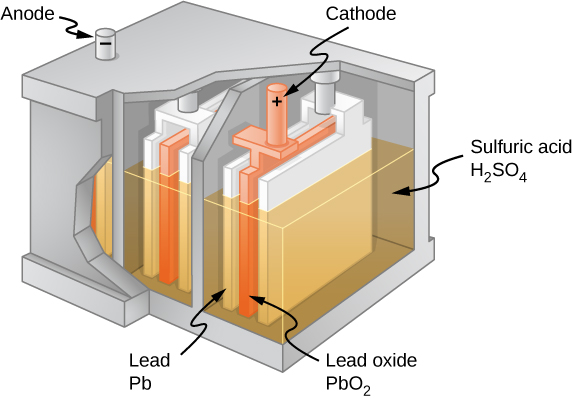# 10.1 Electromotive force  (Page 2/11)

 Page 2 / 11

Positive current flow is useful for most of the circuit analysis in this chapter, but in metallic wires and resistors, electrons contribute the most to current, flowing in the opposite direction of positive current flow. Therefore, it is more realistic to consider the movement of electrons for the analysis of the circuit in [link] . The electrons leave the negative terminal, travel through the lamp, and return to the positive terminal. In order for the emf source to maintain the potential difference between the two terminals, negative charges (electrons) must be moved from the positive terminal to the negative terminal. The emf source acts as a charge pump, moving negative charges from the positive terminal to the negative terminal to maintain the potential difference. This increases the potential energy of the charges and, therefore, the electric potential of the charges.

The force on the negative charge from the electric field is in the opposite direction of the electric field, as shown in [link] . In order for the negative charges to be moved to the negative terminal, work must be done on the negative charges. This requires energy, which comes from chemical reactions in the battery. The potential is kept high on the positive terminal and low on the negative terminal to maintain the potential difference between the two terminals. The emf is equal to the work done on the charge per unit charge $\left(\epsilon =\frac{dW}{dq}\right)$ when there is no current flowing. Since the unit for work is the joule and the unit for charge is the coulomb, the unit for emf is the volt $\left(1\phantom{\rule{0.2em}{0ex}}\text{V}=1\phantom{\rule{0.2em}{0ex}}\text{J/C}\right).$

The terminal voltage     ${V}_{\text{terminal}}$ of a battery is voltage measured across the terminals of the battery when there is no load connected to the terminal. An ideal battery is an emf source that maintains a constant terminal voltage, independent of the current between the two terminals. An ideal battery has no internal resistance, and the terminal voltage is equal to the emf of the battery. In the next section, we will show that a real battery does have internal resistance and the terminal voltage is always less than the emf of the battery.

## The origin of battery potential

The combination of chemicals and the makeup of the terminals in a battery determine its emf. The lead acid battery used in cars and other vehicles is one of the most common combinations of chemicals. [link] shows a single cell (one of six) of this battery. The cathode (positive) terminal of the cell is connected to a lead oxide plate, whereas the anode (negative) terminal is connected to a lead plate. Both plates are immersed in sulfuric acid, the electrolyte for the system.Chemical reactions in a lead-acid cell separate charge, sending negative charge to the anode, which is connected to the lead plates. The lead oxide plates are connected to the positive or cathode terminal of the cell. Sulfuric acid conducts the charge, as well as participates in the chemical reaction.

Knowing a little about how the chemicals in a lead-acid battery interact helps in understanding the potential created by the battery. [link] shows the result of a single chemical reaction. Two electrons are placed on the anode , making it negative, provided that the cathode supplies two electrons. This leaves the cathode positively charged, because it has lost two electrons. In short, a separation of charge has been driven by a chemical reaction.

what is principle of superposition
what are questions that are likely to come out during exam
what is electricity
watt is electricity.
electricity ka full definition with formula
Jyoti
If a point charge is released from rest in a uniform electric field will it follow a field line? Will it do so if the electric field is not uniform?
Maxwell's stress tensor is
Yes
doris
neither vector nor scalar
Anil
if 6.0×10^13 electrons are placed on a metal sphere of charge 9.0micro Coulombs, what is the net charge on the sphere
18.51micro Coulombs
ASHOK
Is it possible to find the magnetic field of a circular loop at the centre by using ampere's law?
Is it possible to find the magnetic field of a circular loop at it's centre?
yes
Brother
The density of a gas of relative molecular mass 28 at a certain temperature is 0.90 K kgmcube.The root mean square speed of the gas molecules at that temperature is 602ms.Assuming that the rate of diffusion of a gas in inversely proportional to the square root of its density,calculate the density of
A hot liquid at 80degree Celsius is added to 600g of the same liquid originally at 10 degree Celsius. when the mixture reaches 30 degree Celsius, what will be the total mass of the liquid?
Under which topic
doris
what is electrostatics
Study of charges which are at rest
himanshu
Explain Kinematics
Two equal positive charges are repelling each other. The force on the charge on the left is 3.0 Newtons. Using your notes on Coulomb's law, and the forces acting on each of the charges, what is the force on the charge on the right?
Using the same two positive charges, the left positive charge is increased so that its charge is 4 times LARGER than the charge on the right. Using your notes on Coulomb's law and changes to the charge, once the charge is increased, what is the new force of repulsion between the two positive charges?
Nya
A mass 'm' is attached to a spring oscillates every 5 second. If the mass is increased by a 5 kg, the period increases by 3 second. Find its initial mass 'm'
a hot water tank containing 50,000g of water is heated by an electric immersion heater rated at 3kilowatt,240volt, calculate the current
what is charge
product of current and time
JaffarBy Michael PittBy OpenStaxBy OpenStaxBy Rylee MinllicBy OpenStaxBy OpenStaxBy OpenStaxBy Cath YuBy Tony PizurBy Richley Crapo